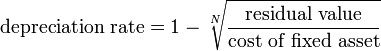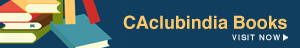Rohit Pandey , 12 January 2015

Hi Friends,

In CA exams we are allowed to use only a basic calculator and not a scientific one. There are certain problems which we find difficult to solve on calculator. Recently, I came accross a forum message posted by Ms. Nidhi stated as under:

Original cost-100000 Scrap value-5000 Useful life-6 yrs Rate-?

Formula given to find the rate: {1-[(scrapvalue/originalcost)^1/n]} how to get the rate ? pls help

/forum/details.asp?mod_id=316603&offset=1#.VLIxK9JySZA

After reading her problem, I decided to write an article to help all aspiring CA students to solve such problem on basic calculator.

If I would have just answered to her message alone then It would have reached to few.

So here you go....

First, I am going to tell you how to calculate any root, and not just square root, on a basic calculator.

Step 1

Take any number say 1234

Step 2

Press 'ROOT' button on calculator 12 (twelve) times.

Step 3

Deduct 1 from the resulting figure. (Press - button and then press 1)

Step 4

Divide the resulting figure by the root you want to calculate.

For eg. If you want to calculate cube root, divide it by 3. If you want to calculate 6th root, then divide it by 6 and likewise.

Step 4

Add back 1 you deducted earlier in Step 3 (Press + button and then press 1)

Step 5

Now you are at final step. You have to do contrary of Step 1.

Press 'x' (MULTIPLY) and then '=' (EQUAL) in combination, twelve times.

For further clarification, first press 'x' then '='. Then again 'x' then '='. Follow this combination of step twelve times. Count 1 when you press '=' and stop when you count till 12.

Now, If you are clear about calculating any root on a basic calculator, you are ready to solve a depreciation problem posted by Ms. Nidhi.

As per her question, Formula for calculating depreciation rate is:Residual Value = Rs. 5000

Cost of Fixed Asset = Rs. 100000

N = 6

So, we have to calculate 6th root of 5000/100000 i.e. 0.05

Step 1

Press ROOT button twelve times.

Resulting figure: 0.99926888743

Step 2

Deduct 1 from Resulting figure in Step 1

Resulting figure: -0.00073111257

Step 3

Divide Resuting figure in Step 2 by 6 (Six)

Resulting figure: -0.00012185209

Step 4

Add 1 to resulting figure in Step 3

Resuting figure: 0.99987814791

Step 5

Now, press 'x' and then '=' in combination, 12 (Twelve) times

Resulting Figure: 0.6070545673

Step 6

Now as per formula of calculating depreciation rateDeduct resulting figure in Step 5 from 1 (One)

{You may put resulting figure in Step 5 in 'M-' and Then, press 1 and put it in 'M+'. Then press 'MR' button. You will get answer by this.)

Hope you like this article on trick for calculating any ROOT on a basic calculator.

You might be finding it difficult to memorize at once. But, when you will practice it 2-3 times, you will get used to it and it shall come easy to you.

By Rohit Pandey

rohitofficial@hotmail.com

Currently working with RPMD & Associates

Rohit Pandey
(Student)
Category Others   Report

8 Likes   151 Shares   60039 Views

### Related Articles### Popular ArticlesCCI Articles